• 概率机器人，打标签的扫描pdf版 对概率机器人学这一新兴领域进行了全面的介绍。概率机器人学依赖统计技术表示信息和进行决策，以容纳当今大多数机器人应用中必然存在的不确定性，是机器人学的一个分支。它依赖统计...
• 概率机器人 Probabilistic Robotics，作者Sebastian Thrun, Wolfram Burgard, Dieter Fox, 2006年高清中英文版本。《概率机器人》对概率机器人学这一新兴领域进行了全面的介绍。概率机器人学依赖统计技术表示信息和...
• 概率机器人的作者塞巴斯蒂安的授课PPT，包括离散滤波，fastslam，卡尔曼滤波，粒子滤波，运动模型，感知模型，slam等等
• 概率机器人 需要的自然知道好书值得拥有 做机器人可以看看
• 概率机器人》对概率机器人学这一新兴领域进行了全面的介绍。概率机器人学依赖统计技术表示信息和进行决策，以容纳当今大多数机器人应用中必然存在的不确定性，是机器人学的一个分支。它依赖统计技术表示信息和制定...
• 对接《概率机器人》中用到的图片，流行的pdf是黑白的，而且比较模糊，这个是高清的彩图，记住只有图，没有内容
• 概率机器人》对概率机器人学这一新兴领域进行了全面的介绍。概率机器人学依赖统计技术表示信息和进行决策，以容纳当今大多数机器人应用中必然存在的不确定性，是机器人学的一个分支。它依赖统计技术表示信息和制定...
• 概率机器人》对概率机器人学这一新兴领域进行了全面的介绍。概率机器人学依赖统计技术表示信息和进行决策，以容纳当今大多数机器人应用中必然存在的不确定性，是机器人学的一个分支。它依赖统计技术表示信息和制定...
• 概率机器人学的中英文版本，本书专庄于算法,对于每种算法,均提供了伪代码、完整的数 学推导、实肣结果及算法优缺点分析;各部分自成体系,并包括了一些概率与统 计的基本知识,且避免使用大过先进的数学技术。本书并...
• 机器人行业圣经——概率机器人（Probabilistic Robotics）高清中文版英文版pdf。由于中文版翻译质量不是特别好，建议中英文对照着看。 本书的内容适用于每一位机器人领域的学生、研究者和技木人员，以及应用统计学...
• 概率机器人》配套的英文版PPT，对ROS内容进行了总结和提炼，便于深入理解和学习，ROS学习者必备的课件。
• 中文版概率机器人，带标签目录。塞巴斯蒂安.特龙经典著作，移动机器人入门教材。 本书对概率机器人学这一新兴领域进行了全面的介绍。概率机器人学依赖统计技术表示信息并进行决策，容纳了当今大多数机器人应用中必然...
• probabilistic robotics概率机器人英文原版，这是机器人学很经典的书籍
• discrete-filters.ppt 概率机器人filter
• 这是概率机器人中文翻译高清版.pdf，挺清晰的挺不错的
• 这是概率机器人.pdf英文原版，挺清晰的，还是很不错的
• 概率机器人-贝叶斯滤波与SLAM技术 贝叶斯状态迭代是概率机器人的基础，理解它对平面slam技术，如gmapping算法原理有很大的帮助。先掌握基础原来，以后再考虑如何进行创新。 提示：写完文章后，目录可以自动生成，...
概率机器人-贝叶斯滤波
贝叶斯状态迭代是概率机器人的基础，理解它对平面slam技术，如gmapping算法原理有很大的帮助。先掌握基础原来，以后再考虑如何进行创新。

文章目录概率机器人-贝叶斯滤波前言一、什么是贝叶斯滤波？1、置信度2、贝叶斯滤波算法二、贝叶斯滤波的推导1.基本概念2.公式推导总结

前言
书中介绍的概率机器人是基于隐马尔可夫模型，或者叫动态贝叶斯网络，即时刻$t$的状态随机地依赖t-1时刻的状态和控制输入$u_t$，测量$z_t$随机地依赖时刻t的状态。这就是贝叶斯滤波的基本假设了。看到这个假设，其实我们会想时刻$t$的状态是如何与前面的东西关联起来的，即那状态是如何更新的？大家可以带着问题进行思考。

一、什么是贝叶斯滤波？
1、置信度
首先介绍置信度的概念，贝叶斯滤波就是置信度的递归计算。
置信度的概念：
概率机器人通过条件概率分布表示置信度，是对给定所有过去的传感器测量和所有过去控制的环境状态的一个后验分布。比如$bel(x_t)=p(x_t|z_{1:t},u_{1:t})$，表示状态变量$x_t$的置信度。
如何理解置信度？
机器人的状态是不能测量的，你说$x_t$发生的可能性是多少，给个概率吧！那不就是以过去测量$z_{1:t}$和所有过去控制$u_{1:t}$为条件，时刻t下状态$x_t$的概率分布。
有了$bel(x_t)$，据说刚执行完控制$u_t$，用$z_{t-1}$计算后验也是有用的。$\overline{bel}(x_t)=p(x_t|z_{1:t-1},u_{1:t})$。该概率被称为预测，是基于以前状态的后验。由$\overline{bel}(x_t)$计算$bel(x_t)$称为测量更新。理解了置信度的概念，那到底怎么进行更新？
2、贝叶斯滤波算法
贝叶斯滤波算法讲的就是测量更新的过程。
伪代码如下：

Algorithm Bayes  filter

for all  $x_t$ do

$\quad \overline{bel}(x_{t-1}) =\int p(x_t \mid u_t,x_{t-1})bel(x_{t-1})dx_{t-1}$

$\quad bel(x_t)=\eta p(z_t\mid x_t)\overline{bel}(x_t)$

end for

return $bel(x_t)$

贝叶斯算法就是一个递归的过程，它分两个部分：第一部分，控制更新。通过基于$x_{t-1}$的置信度和控制输入$u_t$来计算状态$x_t$的置信度。第二部分，测量更新。用已经观测到的测量$z_t$的概率乘以置信度$\overline{bel}(x_t)$。但这样有个问题，对每个后验状态$x_t$都这样做，乘积结果常不是一个概率，它的和可能不为1，所以公式中乘了一个归一化系数。
那这公式写得到底可信度有多少？下面来推导一下。
二、贝叶斯滤波的推导
1.基本概念
条件概率：
$p(x\mid y)=\frac{p(x,y)}{p(y)}=\frac{p(x)p(y)}{p(y)}$
全概率公式：
$p(x)=\sum_y p(x\mid y)p(y)$
贝斯准则：
$p(x\mid y)=\frac{p(y\mid x)p(x)}{p(y)}=\frac{p(y\mid x)p(x)}{\sum_{x'}p(y\mid x')p(x')}$
2.公式推导
\begin{aligned} \overline{bel}(x_{t-1})&=p(x_t\mid z_{1:t-1},u_{1:t})\\ &=\int p(x_t\mid z_{1:t-1},x_{t-1},u_{1:t})p(x_{t-1}\mid z_{1:t-1},u_{1:t})dx_{t-1}\\ &=\int p(x_t\mid x_{t-1},u_{1:t})p(x_{t-1}\mid z_{1:t-1},u_{1:t-1})dx_{t-1} \end{aligned}
因为状态是完整的，当条件中有$x_{t-1}$时可以将$z_{1:t-1}$去掉。又因为控制量$u_t$不能发生在状态$x_{t-1}$前，所以可以近似写成$u_{t-1}$发生时，$x_{t-1}$发生的概率。
用条件概率和贝叶斯准则对$bel(x_t)$进行展开:
\begin{aligned} bel(x_t)&=p(x_t\mid z_{1:t}, u_{1:t})\\ &=\frac{p(z_{1:t}\mid x_t, z_{1:t-1}, u_{1:t}) p(x_t\mid z_{1:t-1}, u_{1:t})}{p(z_{1:t}\mid z_{1:t-1}, u_{1:t})}\\ &=\frac{p(z_{1:t}\mid x_t, u_{1:t}) p(x_t\mid z_{1:t-1}, u_{1:t})}{p(z_{1:t}\mid z_{1:t-1}, u_{1:t})}\\ &=\eta p(z_{1:t}\mid x_t) p(x_t\mid z_{1:t-1}, u_{1:t}) \end{aligned}
还是基于状态完整的假设，条件中状态$x_t$存在时可以将测量状态和控制输入去掉。
至此，公式就推完了。该算法的实现需要三个概率分布：初始置信度$p(x_0)$、测量概率$p(z_t\mid x_t)$和状态转移概率$p(x_t\mid u_t, x_{t-1})$。但是这些概率怎么求，或者如何设定，不在此讨论，本文仅对贝叶斯滤波进行介绍和推导。

总结
机器人及其环境的动态以两种概率法则，即状态转移分布和测量分布的形式为特点。（需要初始置信度、测量概率和状态转移概率） 状态转移分布描述状态如何随时间变化的特征，可能作为机器人控制的效果。测量分布描述测量如何由状态控制的特征，两个分布都是概率性的，导致状态演变和检测固有的不确定性。
贝叶斯滤波是基于隐马尔可夫假设，即过去和未来数据都是独立的。但是未建模因素，概率的不精确性会影响位姿的估计。所以以此原理的位姿估计，在同一位置，估计结果应该会不一样。


展开全文• 状态 环境特征以状态表征，状态是所有会对未来产生影响的机器人及其环境的所有方面因素。 概率生成法则 状态转移概率，根据条件独立，...状态转移概率和测量概率一起描述机器人及其周围环境的动态随机系统。...
状态
环境特征以状态表征，状态是所有会对未来产生影响的机器人及其环境的所有方面因素。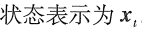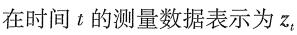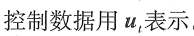概率生成法则
状态转移概率，根据条件独立，可得到：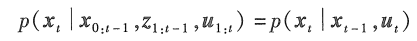它指出了环境状态作为机器人控制ut的函数是如何随着事件变化的。从得到的概率分布公式，可知机器人的环境是随机的。

测量概率，根据条件独立，可得到：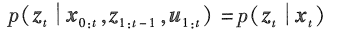它指出测量数据zt由环境状态xt产生，将测量认为是状态的有噪声预测。

隐马尔可夫模型（HMM）/动态贝叶斯网络（DBN）
状态转移概率和测量概率一起描述机器人及其周围环境的动态随机系统。时刻t的状态随机地依赖t-1时刻的状态和控制；时刻t的测量随机地依赖时刻t的状态。这种事件生成模型称为隐马尔可夫模型或动态贝叶斯网络。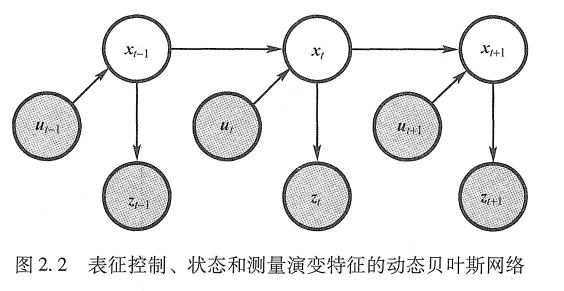马尔可夫假设： 又称完整状态假设，如果知道当前状态，马尔可夫假设设定过去和未来的数据都是独立的。

置信分布
置信度（信息状态）：反映机器人有关环境状态的内部信息。

机器人状态不能直接测量，必须通过数据中推测，因此要从内部置信度识别出真正的状态。概率机器人通过条件概率分布表示置信度。

置信度分布：是以可获得数据为条件的关于状态变量的后验概率。

状态xt的置信度如下：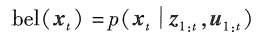这个后验是时刻t下状态xt的概率分布，以过去所有1：t时刻的测量和控制为条件。

在执行完ut之后，综合zt之前计算后验，在概率滤波中，该概率称为预测，表示如下：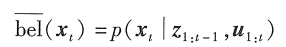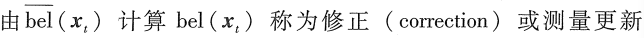**参考书籍：概率机器人

展开全文• py-probabilistic-robotics：概率机器人本地化
• 概率机器人总结——占用栅格地图先实践再推导概率机器人总结——占用栅格地图构建先实践再推导实践过程伪代码分析真代码分析推导过程静态二值贝叶斯滤波 概率机器人总结——占用栅格地图构建先实践再推导 当我将概率...
概率机器人总结——占用栅格地图先实践再推导概率机器人总结——占用栅格地图构建先实践再推导实践过程伪代码分析真代码分析推导过程静态二值贝叶斯滤波
概率机器人总结——占用栅格地图构建先实践再推导
当我将概率机器人看到这里的时候，越发觉将数学理论转到实际应用是一件非常有意思的事情，像我的话很早之前就用过gmapping和amcl这些ros自带的功能包了，但是知其然不知其所以然，看起来很炫酷的操作却不明白其背后的原理是什么，通过这一段时间的学习总结，对此有了一个更加深入的认识，很爽的。
实践过程
伪代码分析
这里我先放上来《概率机器人》中的伪代码其中$m_{i}$就是我们见到的栅格，$l_{t, i}$这个东西叫对数占用概率，由后验概率表示如下：$l_{t, i}=\log \frac{p\left(m_{i} | z_{1, t}, x_{1, t}\right)}{1-p\left(m_{i} | z_{1, t}, x_{1, t}\right)}$当然反过来，当我们更新对数占用概率后可以用其恢复后验概率，如下：$p\left(m_{i} | z_{1, t}, x_{1, t}\right)=1-\frac{1}{1+\exp \left\{l_{t, i}\right\}}$这个让我联想到在视觉SLAM里面用到的李群和李代数的关系，我们回到伪代码，第3-7行表示只对机器人感知范围内的栅格进行更新对数占用概率的更新，不在范围内的不进行跟新，在更新的过程中有一个inverse_sensor_model函数，这个是什么呢？看下面伪代码：看起来会稍微复杂一些，但其实很简单，结合下面这张图解释：首先我们通过观测获得的数据的坐标系肯定是基于我们传感器的，第2-5行可以理解为将其转到世界坐标系下，给定一个传感器观测的范围，也就是上图中的白色区域，第6-12行表示的是，如果我们给定的一个栅格$m_{i}$位于白色区域就返回一个固定值$l_{free}$，如果位于黑色区域就返回一个固定值$l_{occu}$，如果位于灰色区域就返回一个值$l_{0}$，再下面实际的程序里氛围设置为了0.4，0.6和0.5。
真代码分析
PythonRobotics里面关于mapping的Demo太过简单，因此我在知乎上搜到了一个北航小哥写的文章和代码，推导清晰，代码也很简单，一看就懂，先附上链接Grid Mapping 占用栅格地图构建实现，然后粘几段代码过来看一下：
void GridMapper::updateMap ( const sensor_msgs::LaserScanConstPtr& scan,  Pose2d& robot_pose )
{
/* 获取激光的信息 */
const double& ang_min = scan->angle_min;
const double& ang_max = scan->angle_max;
const double& ang_inc = scan->angle_increment;
const double& range_max = scan->range_max;
const double& range_min = scan->range_min;

/* 设置遍历的步长，沿着一条激光线遍历 */
const double& cell_size = map_->getCellSize();
const double inc_step = 1.0 * cell_size;

/* for every laser beam */
for(size_t i = 0; i < scan->ranges.size(); i ++)
{
/* 获取当前beam的距离 */
double R = scan->ranges.at(i);
if(R > range_max || R < range_min)
continue;

/* 沿着激光射线以inc_step步进，更新地图*/
double angle = ang_inc * i + ang_min;
double cangle = cos(angle);
double sangle = sin(angle);
Eigen::Vector2d last_grid(Eigen::Infinity, Eigen::Infinity); //上一步更新的grid位置，防止重复更新
for(double r = 0; r < R + cell_size; r += inc_step)
{
Eigen::Vector2d p_l(
r * cangle,
r * sangle
); //在激光雷达坐标系下的坐标

/* 转换到世界坐标系下 */
Pose2d laser_pose = robot_pose * T_r_l_;
Eigen::Vector2d p_w = laser_pose * p_l;

/* 更新这个grid */
if(p_w == last_grid) //避免重复更新
continue;

updateGrid(p_w, laserInvModel(r, R, cell_size));

last_grid = p_w;
}//for each step
}// for each beam
}

这个是最主要的代码段，这个函数就是从激光的数据结构中遍历每一条激光线，然后以栅格长度的步长去遍历这条激光线上的栅格，然后将各个栅格的坐标从雷达坐标系下转到世界坐标系下，然后逐个更新栅格被占用的后验概率，跟新概率这两个函数如下：
void GridMapper::updateGrid ( const Eigen::Vector2d& grid, const double& pmzx )
{
/* TODO 这个过程写的太低效了 */
double log_bel;
if(  ! map_->getGridLogBel( grid(0), grid(1), log_bel )  ) //获取log的bel
return;
log_bel += log( pmzx / (1.0 - pmzx) ); //更新
map_->setGridLogBel( grid(0), grid(1), log_bel  ); //设置回地图
}


double GridMapper::laserInvModel ( const double& r, const double& R, const double& cell_size )
{
if(r < ( R - 0.5*cell_size) )
return P_free_;

if(r > ( R + 0.5*cell_size) )
return P_prior_;

return P_occ_;
}


这个laserInvModel函数相当于上面伪代码里面的inverse_sensor_model，但是要简单很多，就是判定该栅格距离雷达中心所在位置，而updateGrid函数运行的就是第一段伪代码所示的步骤，对应着看就好了，代码在我电脑上运行效果如下（看上去和gmapping差不多了，gmapping也是不带回环检测的）：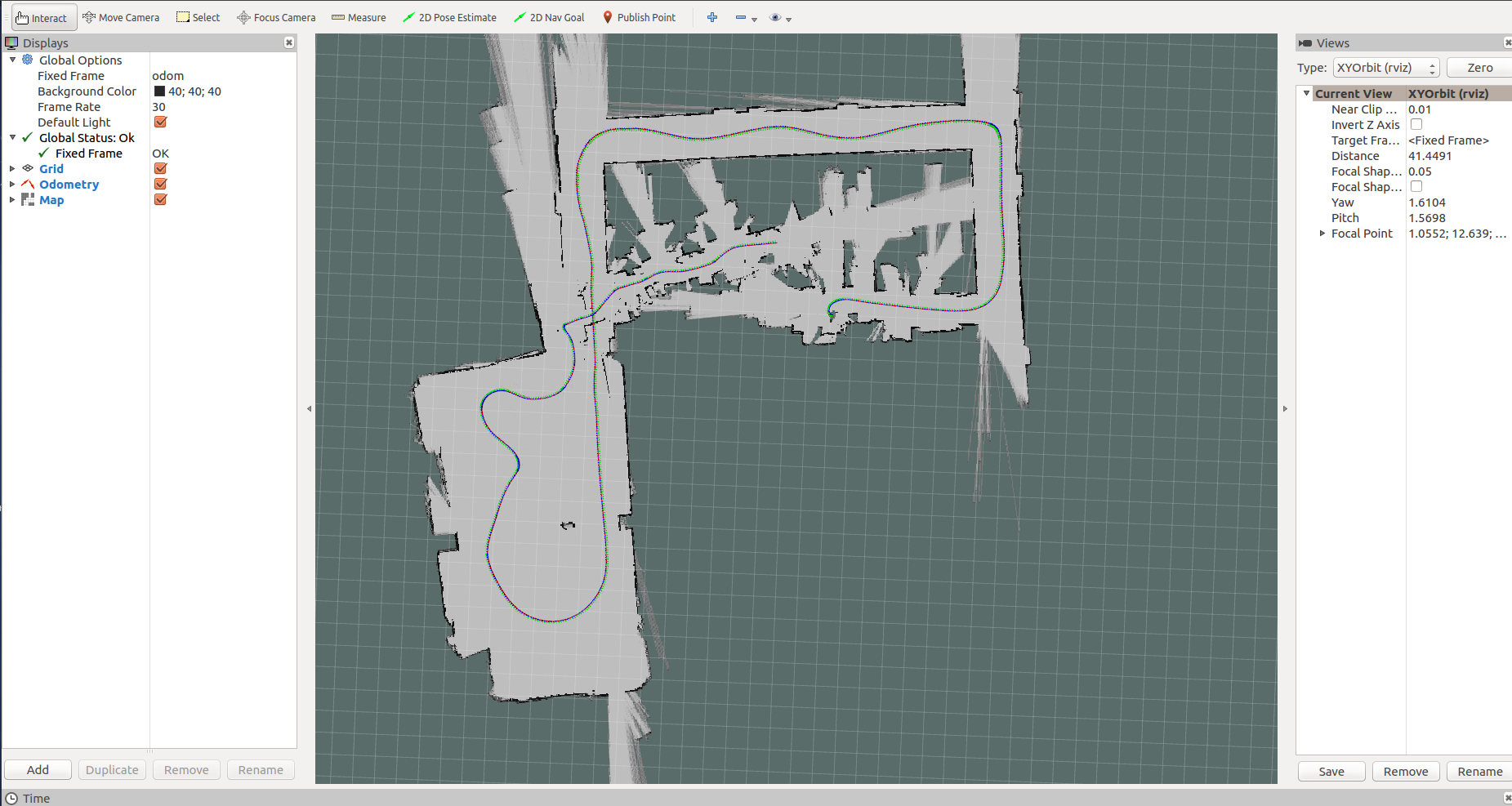推导过程
上面的效果看到了，接下来需要通过推导来看看为什么这么做是好使的，占用栅格地图的构建是基于静态二值贝叶斯滤波实现的。
静态二值贝叶斯滤波
这里基本的贝叶斯滤波方程入手：\begin{aligned} p\left(x | z_{1, t}\right) &=\frac{p\left(z_{t} | x, z_{1, t-1}\right) p\left(x | z_{1, t-1}\right)}{p\left(z_{t} | z_{1, t-1}\right)} \\ &=\frac{p\left(z_{t} | x\right) p\left(x | z_{1, t-1}\right)}{p\left(z_{t} | z_{1, t-1}\right)} \end{aligned}这里$p\left(z_{t} | x\right)$可以称作观测方程，也可以成为似然函数， 而$p\left(x | z_{1, t-1}\right)$可以成为运动方程，也可以成为先验值，因为是静态的所以我们省去了$u_t$，$p\left(z_{t} | z_{1, t-1}\right)$我们一般视为一个常数。现在我们对测量方程再运用一次贝叶斯法则有：$p\left(x | z_{1, t}\right)=\frac{p\left(x | z_{t}\right) p\left(z_{t}\right) p\left(x | z_{1, t-1}\right)}{p(x) p\left(z_{t} | z_{1, t-1}\right)}$这里出现了一个关键的分布$p\left(x | z_{t}\right)$，称为反向观测模型，注意和我们的后验$p\left(x | z_{1, t}\right)$是不同的，那么前向观测模型当然指的是$p\left(z_{t} | x\right)$，那么反向观测模型和前向观测模型有什么区别呢？为什么要这样做？反向观测模型可以理解为设计一个函数根据相机图像来计算门为关着的概率，而前向观测模型指的是描述所有相机图像中显示门为关着的分布更容易些。前者当然要简单些，那为什么普通的贝叶斯滤波不这么做呢? 因为$p\left(z_{t}\right)$我们不知道呀～
然后同理求其对立事件$\neg x$的分布$p\left(\neg x | z_{1, t}\right)=\frac{p\left(\neg x | z_{t}\right) p\left(z_{t}\right) p\left(\neg x | z_{1, t-1}\right)}{p(\neg x) p\left(z_{t} | z_{1, t-1}\right)}$相除得\begin{aligned} \frac{p\left(x | z_{1, t}\right)}{p\left(\neg x | z_{1 ; t}\right)} &=\frac{p\left(x | z_{t}\right)}{p\left(\neg x | z_{t}\right)} \frac{p\left(x | z_{1, t-1}\right)}{p\left(\neg x | z_{1 ; t-1}\right)} \frac{p(\neg x)}{p(x)} \\ &=\frac{p\left(x | z_{t}\right)}{1-p\left(x | z_{t}\right)} \frac{p\left(x | z_{1, t-1}\right)}{1-p\left(x | z_{1, t-1}\right)} \frac{1-p(x)}{p(x)} \end{aligned}因为是二值所以上式才成立，然后我们取对数得\begin{aligned} l_{t}(x) &=\log \frac{p\left(x | z_{t}\right)}{1-p\left(x | z_{t}\right)}+\log \frac{p\left(x | z_{1 ; t-1}\right)}{1-p\left(x | z_{1, t-1}\right)}+\log \frac{1-p(x)}{p(x)} \\ &=\log \frac{p\left(x | z_{t}\right)}{1-p\left(x | z_{t}\right)}-\log \frac{p(x)}{1-p(x)}+l_{t-1}(x) \end{aligned}其中$l_{t}(x)$就是我们定义的概率对数表达式，是我们递归二值后验的一个中间量：$l(x) :=\log \frac{p(x)}{1-p(x)}$然后我们的结论是，二值静态贝叶斯滤波按照如下方式递归：$l_{t}=l_{t-1}+\log \frac{p\left(x | z_{t}\right)}{1-p\left(x | z_{t}\right)}-\log \frac{p(x)}{1-p(x)}$
接下来我们将静态二值贝叶斯滤波应用到占用栅格地图的构建中来：所谓地图构建问题就是根据位置和观测建立地图：$p\left(m | z_{1 : t}, x_{1 : t}\right)$标准的占用栅格方法是按照栅格将其分为一些独立问题：$p\left(m | z_{1, t}, x_{1, t}\right)=\prod_{i} p\left(m_{i} | z_{1, t}, x_{1, t}\right)$然后我们在栅格上建立对数占用概率表达方式：$l_{t, i}=\log \frac{p\left(m_{i} | z_{1, t}, x_{1, t}\right)}{1-p\left(m_{i} | z_{1, t}, x_{1, t}\right)}$然后就利用上述的静态二值贝叶斯滤波进行递归就好了，带有反向观测模型的部分就变成了：$inverse-sensor-model\left(m_{i}, x_{t}, z_{t}\right)=\log \frac{p\left(m_{i} | z_{t}, x_{t}\right)}{1-p\left(m_{i} | z_{t}, x_{t}\right)}$其实就是根据当前的位置和所观测到的情况给栅格赋值，对应了上述的操作，最后我们每迭代一次恢复成将对数占用概率恢复成后验就知道当前栅格是否被占用的概率。
总结完毕，欢迎交流～


展开全文视觉SLAM 占用栅格地图
• 概率机器人的置信分布概率机器人一个主要的概念是置信度(belief),置信度反映了机器人有关环境状态的内部信息，例如，在一些全局坐标系中机器人的位姿可能是xi=(14.12,12.8.450)x_i=(14.12,12.8.45^0),但是因为位姿不...
概率机器人的置信分布

置信度

概率机器人一个主要的概念是置信度(belief),置信度反映了机器人有关环境状态的内部信息，例如，在一些全局坐标系中机器人的位姿可能是xi=(14.12,12.8.450)$x_i=(14.12,12.8.45^0)$,但是因为位姿不能直接测量，通常机器人是不知道自己的位姿的，但是机器人是必须要从数据中推测出其自己的位姿的，因此要从位姿的内部置信度（belief）识别出真正的状态。假设机器人通过条件概率分布表示置信度，对于真实的状态，置信度分布为每一个可能的假设分配一个概率（概率密度值）。置信度分布是以可以获得的数据为条件的关于状态变量的后验概率。用bel(xt)$bel(x_t)$表示，表示状态变量xt$x_t$的置信度，缩写为

bel(xt)=p(xt)|z1:t,u1:t$bel(x_t)=p(x_t)|z_{1:t},u_{1:t}$

这个后验是时刻t$t$下状态xt$x_t$的概率分布，以过去所有的变量x1:t$x_{1:t}$和所有过去的控制u1:t$u_{1:t}$为条件。默认的置信度是综合了测量zt$z_t$后得到的，但是在刚刚执行ut$u_t$之后，综合zt$z_t$之前计算后验是十分有用的，这样的后验就可以表示为：
bel¯¯¯¯(xt)=p(xt|z1:t−1,u1:t)$\overline {bel}(x_t) =p(x_t|z_{1:t-1},u_{1:t})$
在概率滤波的框架下此概率经常被称为预测（prediction），该术语反应了一个事实：bel¯¯¯¯(xt)$\overline{bel}(x_t)$是基于以前状态的后验，在综合时刻t的测量之前预测了时刻t的状态，由bel¯¯¯¯(xt)$\overline{bel}(x_t)$计算bel(xt)$bel(x_t)$称为修正(correction)或测量更新（measurement update）

贝叶斯滤波

计算置信度一般都是贝叶斯滤波算法给出的，伪代码：
Input(bel(xt−1,ut),zt$bel(x_{t-1},u_t),z_t$)
for all xt$x_t$ do
bel¯¯¯¯(xt)=∫P(xt|ut−1)bel(xt−1)$\overline{bel}(x_t)=\int P(x_{t}|u_{t-1})bel(x_{t-1})$
bel(xt)=ηp(zt|xt)bel¯¯¯¯(xt)$bel(x_t) = \eta p(z_{t}|x_t)\overline {bel}(x_t)$
endfor
return bel¯¯¯¯(xt)$\overline {bel}(x_t)$
贝叶斯算法主要是有两个步骤，就是算法第三行，处理控制ut$u_t$通过基于状态xt−1$x_{t-1}$的置信度和控制ut$u_t$来计算xt$x_t$的置信度，也就是说分配给bel¯¯¯¯(xt)$\overline {bel}(x_t)$是通过两个分布：（1）分配给xt−1$x_{t-1}$的置信度（2）由控制u−t$u-t$引起的从状态xt−1$x_{t-1}$到x−t$x-t$的转移概率。这种哦给你更新的步骤叫做控制更新或者预报。
贝叶斯的第二个步骤叫做测量更新，也就是第四行的操作，用已经观测到的测量zt$z_t$的概率乘以置信度bel¯¯¯¯(xt)$\overline{bel}(x_t)$,乘积的结果往往不再是一个概率他们的综合不再为1，因此需要归一化常数 η$\eta$这样就计算出了最后的置信度bel(xt)$bel(x_t)$.
当然为了递归的计算后验置信度，算法是需要一个时刻T=0的初始置信度bel(x0)$bel(x_0)$作为边界条件
贝叶斯滤波的数学推导
贝叶斯滤波的 算法的推导是归纳法说明，必须说明他是由正确的上一步对应的后验p(x_{t-1}|z_{1:t-1},u_{1:t-1)$p(x_{t-1}|z_{1:t-1},u_{1:t-1)$计算后验分布p(xt|z1:t,u1:t)$p(x_t|z_{1:t},u_{1:t})$
(1)推导的第一步涉及到贝叶斯准则：p(x|y,z)=p(y|x,z)p(x|z)P(y|z)$p(x|y,z)=\frac{p(y|x,z)p(x|z)}{P(y|z)}$
计算后验分布：

p(xt|z1:t,u1:t)=p(zt|xt,z1:t−1,u+1:t)p(xt|z1:t−1,u1:t)p(zt|z1:t−1,u1:t)=ηp(zt|xt,z1:t−1,u1:t)p(xt|z1:t−1,u1:t)$p(x_t|z_{1:t},u_{1:t}) = \frac{p(z_t|x_t,z_{1:t-1},u+{1:t})p(x_t|z_{1:t-1},u_{1:t})}{p(z_t|z_{1:t-1},u_{1:t})} \\ \\ =\eta p(z_t|x_t,z_{1:t-1},u_{1:t})p(x_t|z_{1:t-1},u_{1:t})$

那么我们知道η$\eta$是归一化的结果，p(zt|xt,z1:t−1,u1:t)=p(zt|xt|)$p(z_t|x_t,z_{1:t-1},u_{1:t})=p(z_t|x_t|)$ 这是条件独立的表达结果

我们根据前面的知识知道p(xt|z1:t−1,u1:t)=bel¯¯¯¯(xt)$p(x_t|z_{1:t-1},u_{1:t}) = \overline{bel}(x_t)$

所以我们可以得到：bel(xt)=ηp(zt|xt)bel¯¯¯¯(xt−1)$bel(x_t)= \eta p(z_t|x_t)\overline{bel}(x_{t-1})$

然后可以根据是离散情况还是连续情况进行推广贝叶斯滤波，首先我们需要了解一下全概率公式：
离散情况： p(x)=∑p(x|y)p(y)$p(x)= \sum p(x|y)p(y)$
连续情况：p(x)=∫p(x|y)p(y)dy$p(x) = \int p(x|y)p(y)dy$

所以首先对于连续情况下的贝叶斯滤波的一个推广：

bel¯¯¯¯(xt)=p(xt|z1:t−1,u1:t)=∫p(xt|xt−1,z1:t−1,u1:t)p(xt−1|z1:t−1,u1:t)dxt−1$\overline {bel}(x_t) =p(x_t|z_{1:t-1},u_{1:t}) = \int p(x_t|x_{t-1},z_{1:t-1},u_{1:t}) p(x_{t-1}|z_{1:t-1},u_{1:t})dx_{t-1}$
展开全文• 概率机器人中文版和对应的ppt课件，需要的朋友可以看下。人工智能
• 《Probabilistic Robotics》中文译名为《概率机器人》 感谢我的导师在研究生阶段开设了这门课程，带领我们简单的过了一下这本书。 相关资料：http://ais.informatik.uni-freiburg.de/teaching/ss16/robotics/ ...
• 第一章 绪论 1.1 机器人学中的不确定性 机器人必须能接纳客观世界中存在的大量的不...概率机器人学致力于研究机器人感知和行为的不确定性。概率机器人的主要思想是利用概率理论的运算去明确地表示这种不确定性。 1.3...